• 1
•
•
•
•
•
•
•
1
Share

# UNIVERSAL GRAVITATIONAL FORCE

Today we are going to talk about the concept of universal laws of Gravitation. various space agencies launch their satellites by knowing the concept of Gravitation. It means today’s classical technologies are based on the concept of Gravitation.

All the credit for this outstanding work goes to sir Isaac Newton, it is believed that Newton found the concept of Gravitation, when, once he had sat under an apple tree, and suddenly an apple fall from a tree on him.Gravitation is part of classical mechanics and was formulated in Newton’s work Philosophiae Naturalis Principia Mathematica (simply Principia Mathematica), and first published on 5 July 1687.
Now takes a look at these famous laws Newton laws of universal gravitation usually states that:

Every particle attracts other particles in this universe with a force that is directly proportional to the product of their respective masses and inversely proportional to the square of the shortest distance between their centers.

This is a general law derived from the empirical observations, and Newton called this observation inductive reasoning.

And in today’s language, this law is stated as, every point mass attracts every other point mass with a force acting along with the adjoining line, which joins their centers.
This force is directly proportional to the product of their respective masses and inversely proportional to the square of the shortest distance between their centers.

The equation of gravitational force thus takes the form: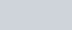Where F is the force acting between them, m1 and m2 are masses and r is the distance between their centers, and G is the proportionality constant called universal gravitational constant.

Newton only combined this formula with his observations. So here we are going to give a mathematical process of deriving this formula, which is as below:

## DERIVATION FOR UNIVERSAL GRAVITATIONAL FORCE

We know that planets revolve in a circular orbit around the sun. [In reality, they revolve in an elliptical orbit, but here we take circular orbit for mathematical reference]
If planets revolve in circular orbit then their centripetal force is given as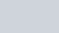Where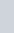is centripetal acceleration.
Then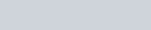Then putting values in centripetal acceleration we get-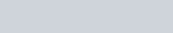And according to the Kepler third laws planetary motion, we know that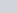is proportional to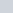Then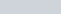Where C is constant  Then putting above acceleration formula in
Below formula-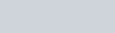Now put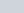in place of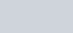Now here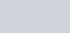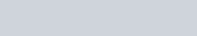And by this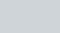, i.e. Constant.
Then putting the value ofin the formula of force. We get-M is the mass of the bigger objects around planets is revolving. This is the required UNIVERSAL GRAVITATIONAL FORCE.